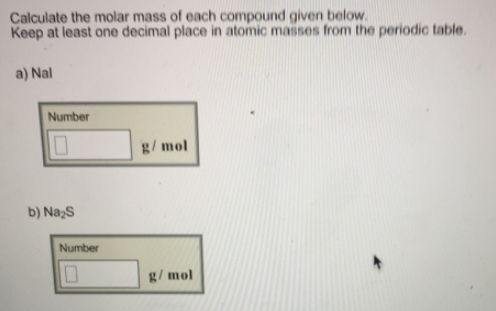# Problem: Calculate the molar mass of each compound given below. Keep at least one decimal place in atomic masses from the periodic table. a) Nal b) Na2S

###### FREE Expert Solution
96% (361 ratings)###### Problem Details

Calculate the molar mass of each compound given below.

Keep at least one decimal place in atomic masses from the periodic table.

a) Nal

b) Na2S